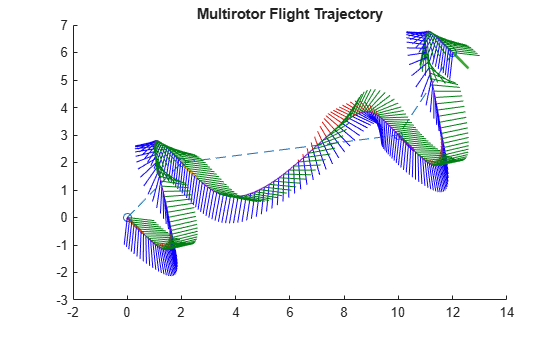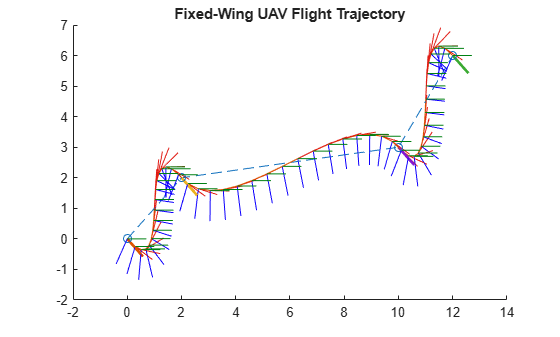# query

Get UAV motion vectors at timestamps

Since R2022b

## Syntax

``motions = query(traj,timestamps)``

## Description

example

````motions = query(traj,timestamps)` gets the UAV motion vectors `motions` from the trajectory `traj` at the timestamps `timestamps`.```

## Examples

collapse all

Create a set of waypoints for both the multirotor and the fixed-wing UAV to follow.

```wpts = [0 0 0; 2 2 -2; 10 10 -3; 12 12 -6]; numwpts = size(wpts);```

Specify additional trajectory information, such as desired velocities, accelerations, jerks, snaps, and yaws, as well as start time, an end time, and times of arrival.

```vels = 2*ones(numwpts); accs = ones(numwpts); jerks = zeros(numwpts); snaps = zeros(numwpts); yaws = zeros(1,numwpts(1)); starttime = 0; endtime = 8; toas = linspace(starttime,endtime,numwpts(1));```

Use the trajectory information to create the flight trajectories for the multirotor and the fixed-wing UAVs. Query and display the trajectories.

```mrft = multirotorFlightTrajectory(wpts,vels,accs,jerks,snaps,yaws,toas); fwft = fixedwingFlightTrajectory(wpts,vels,toas); query(mrft,1:4)```
```ans = 4×16 1.6184 1.6184 0.7520 -0.0243 -0.0243 -2.8758 -4.6045 -4.6045 -9.1669 0.9863 -0.1179 0.1147 -0.0137 0.0732 -0.0732 -0.0000 1.0236 1.0236 -2.7807 0.5482 0.5482 -0.6198 3.9704 3.9704 8.6424 0.7429 0.5559 -0.2987 -0.2235 -1.3257 1.3257 0 2.7277 2.7277 -1.2947 2.4069 2.4069 2.1026 1.7442 1.7442 -1.3857 0.9941 0.0770 -0.0761 -0.0059 0.1950 -0.1950 0 6.4028 6.4028 -2.0972 4.4609 4.4609 -3.8447 -1.1875 -1.1875 -1.1875 0.9971 -0.0537 0.0534 -0.0029 -1.2364 1.2364 -0.0000 ```
`query(fwft,1:4)`
```ans = 4×16 0.9453 0.9453 -0.3203 0.2422 0.2422 -1.8672 -0.7031 -0.7031 -1.5469 0.7098 -0.2450 0.5914 0.2940 -0.4683 0.4683 0.0000 1.1875 1.1875 -2.1875 0.5938 0.5938 -1.0938 1.4063 1.4062 3.0938 0.8287 -0.1692 0.4085 0.3432 1.7750 -1.7750 0 2.7813 2.7812 -1.6055 2.6563 2.6562 0.4414 1.6875 1.6875 -4.0078 0.9223 0.0224 -0.0540 0.3820 -0.7962 0.7962 -0.0000 6.0000 6.0000 -2.5000 3.5000 3.5000 -1.5625 -0.0000 -0.0000 0.0000 0.9131 -0.0583 0.1407 0.3782 -0.0000 -0.0000 0.0000 ```

Visualize both the multirotor flight trajectory and the fixed-wing flight trajectory.

```ax = show(mrft,NumSamples=200); title("Multirotor Flight Trajectory") view([0 0])``````show(fwft,NumSamples=50); title("Fixed-Wing UAV Flight Trajectory") view([0 0])```## Input Arguments

collapse all

Flight trajectory, specified as a `multirotorFlightTrajectory` or `fixedwingFlightTrajectory` object.

Timestamps, specified as an N-element vector containing time values, in seconds. N is the total number of specified timestamps.

## Output Arguments

collapse all

Motion vectors, returned as an N-by-16 matrix, where each row contains the motion vector of the UAV, [X Y Z VX VY VZ AX AY AZ QW QX QY QZ P Q R], at the time specified by the corresponding element of the `timestamps` vector. N is the total number of specified timestamps.

• X, Y, and Z are positions.

• VX, VY, and VZ are velocities.

• AX, AY, and AZ are accelerations.

• QW, QX, QY, and QZ, are quaternions for rotations from the local north-east-down (NED) reference frame to the body frame.

• P, Q, and R are angular velocities along local the NED reference frame axes.

All motion values are expressed in the local NED frame.

For timestamps outside the `StartTime` and `EndTime` of the specified trajectory object, the values of the corresponding motion vector row are `NaN`.

## Version History

Introduced in R2022b